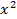Quadratic equations are equations of the form a + bx + c = 0.To solve a quadratic equation we must firstly make sure our equation equals zero. For

instance if + 8x + 8 = -7 we must bring -7 to the other side of our equation so as itequals 0 i.e. + 8x + 15 = 0Once our equation equals 0 it can be solved by factorisation. We let both of our factorised

terms equal 0 and solve for x

+ x - 42 = 0 3 + 19x + 26 = 0(x - 6)(x + 7) = 0 ...